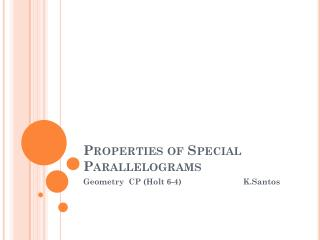DownloadDownload PresentationProperties of Special Parallelograms

Properties of Special Parallelograms

Télécharger la présentationProperties of Special Parallelograms

- - - - - - - - - - - - - - - - - - - - - - - - - - - E N D - - - - - - - - - - - - - - - - - - - - - - - - - - -
Presentation Transcript

1. Properties of Special Parallelograms Geometry CP (Holt 6-4) K.Santos

2. Special Parallelograms Special Parallelograms: Rectangle—a quadrilateral with 4 right angles Rhombus—a quadrilateral with 4 congruent sides Square—a quadrilateral with 4 right angles & 4 congruent sides

3. Theorem 6-4-1 If a quadrilateral is a rectangle, then it is a parallelogram. A B C D ABCD is a parallelogram

4. Theorem 6-4-2 If a parallelogram is a rectangle (square) then its diagonals are congruent. A B D C Given: ABCD is a rectangle Then:

5. Theorem 6-4-3 If a quadrilateral is a rhombus, then it is a parallelogram. A B D C ABCD is a parallelogram

6. Theorem 6-4-4 If a parallelogram is a rhombus (or square), then its diagonals are perpendicular A B D C Given: ABCD is a rhombus Then: ⟘

7. Theorem 6-4-5 If a parallelogram is a rhombus (square) then each diagonal bisects a pair of opposite angles. A B D C Given: ABCD is a rhombus Then: <DAB and <DCB are bisected (by <ABC and <ADC are bisected (by )

8. Example: Rhombus Find the missing angles: 40 Diagonals are perpendicular Diagonals bisect the angles

9. Example: Rectangle 40 Watch for isosceles triangles

10. Example: Square Diagonals perpendicular Diagonals bisect right angles

11. Example: One diagonal of a rectangle has length 8x + 2. The other diagonal has length 5x + 11. Find the length of each diagonal. Diagonals of a rectangle are congruent 8x + 2 = 5x + 11 3x + 2 = 11 3x = 9 x = 3 8x + 2 = 8(3) + 2 = 26 5x + 11 = 5(3) + 11 = 26 Both diagonals measure 26.

12. Example: S T RSTV is a rhombus. Find each measure. ST = 4x + 7 and SR = 9x -13. R V All sides congruent 9x – 13= 4x + 7 5x -13 = 7 5x = 20 x = 4 ST = 4(4) + 7 = 23 SR = 9(4) – 13 = 23

13. Example: S T RSTV is a rhombus. m<TSW = y+2 and m<SWT = 2y+10 Find y and m<WSR. W R V Diagonals of rhombus are perpendicular m<SWT = 90 2y + 10 = 90 2y = 80 y = 40 Diagonal bisected the angle m<WSR = y + 2 = 40 + 2 = 42